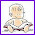### Clock face problem

Divide the face of the clock into three parts with two lines so that the sum of the numbers in the three parts are equal.

Let's first understand the problem. We need to draw lines into the clock face. It doesn't say the lines need to end in the center or go through the center. The lines could seemingly go many various ways.

Again, to help a student who can't even get started, just tell them to draw some lines into the clock face. Here's one example:Of course this is not a solution since it does not fulfill the condition that the sum of the numbers in the three parts needs to be equal. But it gets you started. You have some lines, so you can ask the student to add the numbers in the three parts and verify the sums are NOT equal.

How do we make the sums to be equal? Just random trying would take a long time.

The KEY to this problem is that since the sum in each of the three parts is equal, if you add those three sums, you will get the sum of all the numbers in the clock face. Thus, we can find out the partial sum by computing the total sum and dividing by 3.

1 + 2 + 3 + 4 + 5 + 6 + 7 + 8 + 9 + 10 + 11 + 12 = 78. 78 ÷ 3 = 26.

So the sum of the numbers in each part has to be 26. After this key point, solving this problem is fairly easy.

Now, we need to find numbers on the clock face that will add up to 26. Again, trial and error will probably produce the result fairly soon. But we can use some thinking too:

Let's consider first the largest number on the clock, 12. What other numbers should go with 12 to make 26?
We can add lots of its neigbors to it and try.

12 + 1 + 2 + 3 + 4 + 5 = 27 so just adding numbers from 1 on in order won't work.

That means we might need to couple 12 and 11.

12 + 11 = 23. Need three more. So... 12 + 11 + 1 + 2 will give 26. That's the one part that we can separate with one line.

Then continuing from the larger ones, 10 + 9 + 8 = 27. This is too much. Need another approach.

But let's draw lines across the clock face so that 10 can be joined with some smaller numbers. You will see soon that 10 + 9 + 3 + 4 = 26.The last ones left are 5 + 6 + 7 + 8 = 26.

Now we can try hunt for another solution. Is there one?

Note that we can only draw two lines. You can't do this in very many different ways. You can draw two lines across the clock face, or perhaps draw one line across and another from the circumference to the first line, as in my first picture. In the one solution we've found, the two parts consisted of neighboring numbers and the one middle part did not. In either case, we need to use some neighboring numbers to make up the sums.

When you consider the numbers from 1 to 12 on the clock face, the only neighboring numbers that add up to 26 are the ones we used: 12 + 11 + 1 + 2 and 5 + 6 + 7 + 8. So we have to use at least one of those sets for the one part. If you try it out, you will quickly note no other solutions are possible than the one found above.

This may not be considered a totally rigorous argument by mathematical standards, but it is important for students to become convinced that there are no other solutions. Convincing others of the same is the first step towards mathematical PROOF.mathmom said…
Maria,

I solved it like you suggested (figured out that I needed 26's and found them by trial and error).

My 11yo son actually had a more elegant approach which he describe as using "the rule of rainbows". What he did was first add the numbers from 1 to 12 using Gauss's Method. He found that there were 6 "rainbows" each of which summed to 13. If he could draw lines on the clock so that the four numbers from 2 of the "rainbows" were in each segment of the clock, he'd be done. And it all falls out beautifully that way. 1+12 and 2+11 go in one segment, then 3+10 and 4+12 in the next, and finally 5+8 and 6+7 in the last. (I hope that's clear enough without drawing pictures.)

Neither of us tried to prove whether or not our solution is unique.Michelle G. said…
mathmom, I'm not sure I understand part your "rule of rainbows" explanation. How do 4+12 end up in the rainbow? Or did you mean to say 4+9?mathmom said…
Yes, good catch. I meant 4+9.